# Word 中用 EQ 域编辑数学公式详解

## 一、有关域的几个名词

1、域：指导 Word 在文档中自动插入文字、图形、页码和其它资料的一组代码。

2、域开关：在使用域时，引发特定操作的特殊说明，一般是指添至域中用于修饰结果的选项。

3、域名：域的名称，如 EQ 域、TIME 域等。

4、域记号：一对大括号 {}。注意不能直接用键盘输入，应该用后面介绍的“插入空白域”的方法来实现。

5、域的两种显示方式：一种是以域代码方式显示，一种以域结果方式显示。以 "Time" 域为例，它以域代码方式显示时是这样的：{TIME \*MERGEFORMAT}，它以域结果方式显示是这样的：14:18。

## 二、与域有关的快捷键

1、在文档中插入空白域：按Ctrl+F9键（也可以用命令"插入→域"来实现）。

2、在域代码和域结果之间切换：按Shift+F9键。

3、更新选择的域：按F9键。

4、取消某个域的链接：先选中该域，再按Ctrl+Shift+F9键，这时域结果将以平常文本的形式显示出来。

5、转到前一个域：按Shift+F11键；转到后一个域：按F11键。

6、锁定域：按Ctrl+F11键。例如，在文档中插入Time域，在打印文档时会自动更新该域，如果希望保持插入的时间值，只要在打印之间锁定该域就可以了。解除域的锁定：按Ctrl+Shift+F11键。

## 三、“域”的基本操作

“域”的操作包括对话框操作和热键代码操作两种方式，下面以“$$2\frac{2}{3}$$”的录入过程来介绍“域”的基本操作方法：

## 四、“域”操作中应注意的问题

### 2、在“域”代码输入中应注意以下几点

（1）字母的大小写不区分，但“域”的控制符部分（如上例中的EQ、f、括号、逗号等）必须在英文半角状态下输入，内容部分则无此限制，可以是汉字、特殊符号等。

（2）“域”名和第一个开关之间至少要有一个空格。

（3）“域”内容部分仍可以像文本内容一样选择字体、字号等。

（4）当“域”代码内容很长时，不能强行换行。

（5）不能删除Windows中的Symbol字库文件，否则“域”操作所需要的某些符号就会发生改变。

## 五、EQ域的基本使用格式

{EQ Switches}，其中Switches用于指定如何使用其后跟随的括号中的元素建立公式，还可以用适当的开关选项来进行修改。EQ域的开关特别多，特别复杂，合理的组合可以产生各种各样的公式，下面我们来详细介绍各种开关的作用及使用方法及事例。

## 六、EQ域的开关及选项意义

### 1．数组开关：\a()，可以在文档中绘制一个二维数组

\al 列内左对齐。\ac 列内居中对齐。\ar 列内右对齐。\con元素排成n列（默认值为1）。\vsn行间增加n磅的垂直间距。\hsn列间增加 n 磅的水平间距。

【例1】我们要在文档中输入今年10月份的月历，可以插入如下域代码： {eq \a\ac\co7\vs2\hs10(日,一,二,三,四,五,六,,1,2,3,4,5,6,7,8,9,10,11,12,13,14,15,16,17,18,19,20,21,22,23,24,25,26,27,28,29,30,31)}，显示结果如下所示：

【例2】在文档中插入如下域代码： { EQ \a\ac\co3\vs4\hs9(姓名,年龄,性别,张强,24,男,英子,18,女,姚兴云,29,男) }，其显示结果如下图所示：

【例3】{ EQ \a\al\co2\vs3\hs3(Axy,Bxy,A,B) }，该域的含义是将域代码括号中的数组按行顺序排列成3列，列内居中对齐，行间增加9磅的垂直间距，列间增加9磅的水平间距。其显示结果如下图所示：

$$\begin{array}{*{20}{c}} {Axy}&{Bxy}\\ A&B \end{array}$$

### 2．括号开关：\b()，用括号括住单个元素

\lc\c 左括号使用字符 c 。\rc\c 右括号使用字符 c 。\bc\c 左右括号都使用指定的字符 c 。

【例4】要输入一个三阶行列式，可以输入如下域代码：{eq \b\bc\|(\a\ac\co3\vs4\hs4(y,y+1,y-1,2,y,y+4,y+6,y-2,y))}，显示结果如下图所示：

$$\left| {\begin{array}{*{20}{c}} y&{y + 1}&{y - 1}\\ 2&y&{y + 4}\\ {y + 6}&{y - 2}&y \end{array}} \right|$$

【例5】域代码：{ EQ \b\bc\[(\a\ac\co3\vs9\hs9(x,1+x,1-x,1,x-1,x,1-x))}的显示结果结果如下图所示：

$$\left[ {\begin{array}{*{20}{c}} x&{1 + x}&{1 - x}\\ 1&{x - 1}&x\\ {1 - x}&{}&{} \end{array}} \right]$$

【例6】域代码{ EQ \b\bc\{(\r(5,x2+y2)) }的显示结果如下图所示：

$$\left\{ {\sqrt{{x2 + y2}}} \right\}$$

### 3．位移开关：\d()，将下一个字符向左或右移动指定磅数

\fon()右边n磅。\ban()左边n磅。\li()为下一个字符前的空白添加下划线。

【例7】文字及域代码“我距后面20磅{ EQ \d\fo20\li() } 我距前面20磅”的显示结果为：

“我距后面20磅_________我距前面20磅”

### 4．分数开关：\f(,)：可用于创建分数，分子分母分别在分数线上下居中

【例8】文字和域代码“班平均成绩={ EQ \f（全班总成绩,全班总人数） } ”的显示结果如下图所示：

【例9】{EQ \f(4,RateX) + \f(8,RateX) = \f(12,RateX)}的显示结果如下图所示：

$$\frac{4}{{RateX}} + \frac{8}{{RateX}} = \frac{{12}}{{RateX}}$$

### 5．列表开关：\l()，将多个值组成一个列表，列表可作为单个元素使用

【例10】域代码a=α（{EQ \l(i,j,r,s)}）的显示结果为：

a=α（i,j,r,s ）

【例11】{ EQ \l(A,B,C,D,E)}显示为：

A,B,C,D,E

### 6．重叠开关：\o()，将每个后续元素打印在前一元素之上

\al 左对齐，\ac 居中（默认值），\ar 右对齐。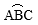### 7．根号开关：\r(,)，使用一个或两个元素绘制根号

【例12】如果我们要建立一个既有根式又有分式的数学方程式，本来非常难，现在好办了。输入域代码：{eq \r(3,1+2x)}={eq \f(1,1-x)}（等号前后各是一个EQ域），其显示结果如下：

$$\sqrt{{1 + 2x}} = \frac{1}{{1 - x}}$$

【例13】域代码{ EQ \r(1+tg2x)}的显示结果如下所示：

$$\sqrt {1 + tg2x}$$

### 8．上标或下标开关：\s()，设置上下标可将一个或多个元素设置为上标或下标

\ain()在段落一行之上添加由 n 指定的磅数的空白。

\upn()将单个元素相对相邻文字上移由 n 指定的磅数。默认值为 2 磅。

\din()在段落一行之下添加由 n 指定的磅数的空白。

\don()将单个元素相对相邻文字下移由 n 指定的磅数。默认值为 2 磅。

【例14】

（1）域代码S{EQ \s\do6(m,n)}= A{EQ \s\do6(m,s)}×B{EQ \s\do6(s,n)}，其显示结果如下图所示：

$$S_n^m = A_s^m \times B_n^s$$

（2）域代码 y = a{ EQ \s\up6(x-1) }的显示结果如下图所示：

y = ax-1

【例15】{ EQ \s\up8(AB)\s\do8(2) } 的显示结果如下图所示：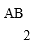### 9．方框开关：\x()，在元素四周绘制边框

\to 元素之上，\bo 元素之下，\le 元素左侧，\ri 元素右侧。

【例16】（1）域代码{EQ \x\to\bo(x2+y2=R2)}显示结果如下：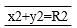（2）域代码{ EQ \x(x2+y2=R2)}显示结果如下：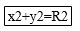（3）域代码{ EQ \x\le\ri(10) } 显示结果如下：

$$\left| {10} \right|$$

### 10．积分开关：\i(,,)，使用指定的符号或默认符号及三个元素创建积分

\su 符号更改为大写的 ∑ 并生成求和公式。

\pr 符号更改为大写的 Π 并生成求积公式。

\in 创建行内格式，积分限不在符号的上下，而在符号之右。

\fc\c 将符号设置为固定高度的字符 c。

\vc\c 将符号设置为可变高度的字符 c。符号高度与第三个元素高度一致。

【例17】（1）域代码{ EQ \i\pr(i=1,n,Si×Ti） }

$$\prod\limits_{i = 1}^n {Si \times Ti}$$

（2）域代码{ EQ \i\in(a,b,sinxdx) }

$$\int_a^b {\sin xdx}$$

（3）{ EQ \i\fc\$(a,b,sinxdx)} $$\mathop \limits_a^b \sin xdx$$ （4）域代码{EQ \i\fc\$(a,b,\f(1,sinx)dx)}

$$\mathop \limits_a^b \frac{1}{{\sin x}}dx$$

（5）域代码{ EQ \i\su(1,5,3) }

$$\sum\limits_1^5 3$$

（6）域代码{ EQ \i\vc\J(a,b,sinxdx)}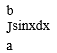（7）域代码{ EQ \i\vc\J(a,b,\f(1,sinx)dx) }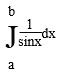## 七、EQ域实例

【例1】我们要创建如下的数学二元一次方程组：先写出第一个方程的域代码：{EQ \r(3, x )+ \r(2, x ) = \r(6, y )}，再写出第二个方程的域代码：{\f( 2 , x ) + \f( 5 , y ) = \f( 10 , x )}。

{ EQ \a\al\col(\r(3, x )+ \r(2, x ) = \r(6, y ), \f( 2 , x ) + \f( 5 , y ) = \f( 10 , x ))}

$$\begin{array}{l} \sqrt{x} + \sqrt{x} = \sqrt{y}\\ \frac{2}{x} + \frac{5}{y} = \frac{{10}}{x} \end{array}$$

{ EQ \b\lc\{(\a\al\col(\r(3, x )+ \r(2, x ) = \r(6, y ), \f( 2 , x ) + \f( 5 , y ) = \f( 10 , x )))}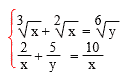{ EQ \b\lc\{(\a\al\col(\r(3, x )+ \r(2, x ) = \r(6, y )……（1）, \f( 2 , x ) + \f( 5 , y ) = \f( 10 , x )……（2）))}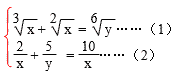{ EQ \b\lc\{(\a\al\co2(\r(3, x )+ \r(2, x ) = \r(6, y ),……（1）, \f( 2 , x ) + \f( 5 , y ) = \f( 10 , x ),……（2）))}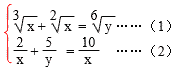【例2】创建如下的积分算式：

{EQ \i(200,100,\f(\r(2,x),5)) dx}，最终的显示结果如图所示：

$$\int\limits_{100}^{200} {\frac{{\sqrt{x}}}{5}dx}$$

$$\int_a^b {\frac{1}{{1 + x}}dx = \left[ {\ln x} \right]_a^b}$$

$$\int_a^b {dx\int_a^x {f(x,y)} dy = } \int_a^b {dy\int_y^b {f(x,y)} dx}$$

$${e^{\lambda x}}\left[ {{P_1}\frac{{{e^{i\omega x}} + {e^{ - i\omega x}}}}{2} + {P_n}\frac{{{e^{i\omega x}} - {e^{ - i\omega x}}}}{2}} \right]$$

$$\left\{ \begin{array}{l} x = \frac{{12}}{{17}}{e^{ - \frac{7}{5}t}} + \frac{5}{{17}}{e^{2t}} + \frac{3}{7}t - \frac{1}{{49}}\\ y = \frac{{18}}{{17}}{e^{ - \frac{7}{5}t}} - \frac{1}{{17}}{e^{2t}} + \frac{1}{2}{e^{ - t}} - \frac{{26}}{{49}} \end{array} \right.$$

2006 - 2023，推荐分辨率1024*768以上，推荐浏览器Chrome、Edge等现代浏览器，截止2021年12月5日的访问次数：1872万9823 站长邮箱